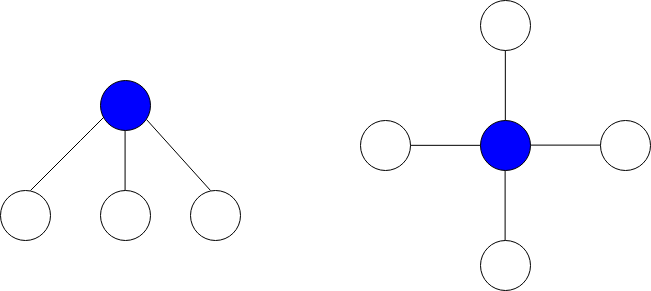2497. Maximum Star Sum of a Graph
Medium
262
29

There is an undirected graph consisting of `n` nodes numbered from `0` to `n - 1`. You are given a 0-indexed integer array `vals` of length `n` where `vals[i]` denotes the value of the `ith` node.

You are also given a 2D integer array `edges` where `edges[i] = [ai, bi]` denotes that there exists an undirected edge connecting nodes `ai` and `bi.`

A star graph is a subgraph of the given graph having a center node containing `0` or more neighbors. In other words, it is a subset of edges of the given graph such that there exists a common node for all edges.

The image below shows star graphs with `3` and `4` neighbors respectively, centered at the blue node.The star sum is the sum of the values of all the nodes present in the star graph.

Given an integer `k`, return the maximum star sum of a star graph containing at most `k` edges.

Example 1:```Input: vals = [1,2,3,4,10,-10,-20], edges = [[0,1],[1,2],[1,3],[3,4],[3,5],[3,6]], k = 2
Output: 16
Explanation: The above diagram represents the input graph.
The star graph with the maximum star sum is denoted by blue. It is centered at 3 and includes its neighbors 1 and 4.
It can be shown it is not possible to get a star graph with a sum greater than 16.
```

Example 2:

```Input: vals = [-5], edges = [], k = 0
Output: -5
Explanation: There is only one possible star graph, which is node 0 itself.
Hence, we return -5.
```

Constraints:

• `n == vals.length`
• `1 <= n <= 105`
• `-104 <= vals[i] <= 104`
• `0 <= edges.length <= min(n * (n - 1) / 2``, 105)`
• `edges[i].length == 2`
• `0 <= ai, bi <= n - 1`
• `ai != bi`
• `0 <= k <= n - 1`
Accepted
12.8K
Submissions
33.3K
Acceptance Rate
38.4%

Seen this question in a real interview before?
1/4
Yes
No

Discussion (0)

Related Topics